# Piecewise smooth path

## Definition

Let$M$ be a differential manifold and$p,q \in M$ be two (not necessarily distinct) points. A piecewise smooth path$\omega$ from$p$ to$q$ is a map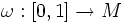$\omega:[0,1] \to M$ such that:

• There exists a partition$0 = t_0 < t_1 < \ldots < t_k = 1$ such that$\omega$ restricted to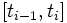$[t_{i-1},t_i]$ is smooth.
•$\omega(0) = p$ and$\omega(1) = q$

## Notions

### Set of all piecewise smooth paths between two points

Further information: Path space of a manifold

The set of all piecewise smooth paths in a differential manifold$M$ between two points$p,q \in M$ is denoted as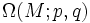$\Omega(M;p,q)$, sometimes simply as$\Omega(M)$ or simply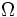$\Omega$.

Fill this in later

### Tangent space

From the infinite-dimensional manifold definition, the following definition emerges for the tangent space to a piecewise smooth curve with respect to the path space.

The tangent space of a path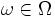$\omega \in \Omega$ is defined as the vector space of all piecewise smooth vector fields$W$ along$\omega$ for which$W(0) = W(1) = 0$. In other words, it is an infinitesimal change in the path, with no change occurring at the endpoints.